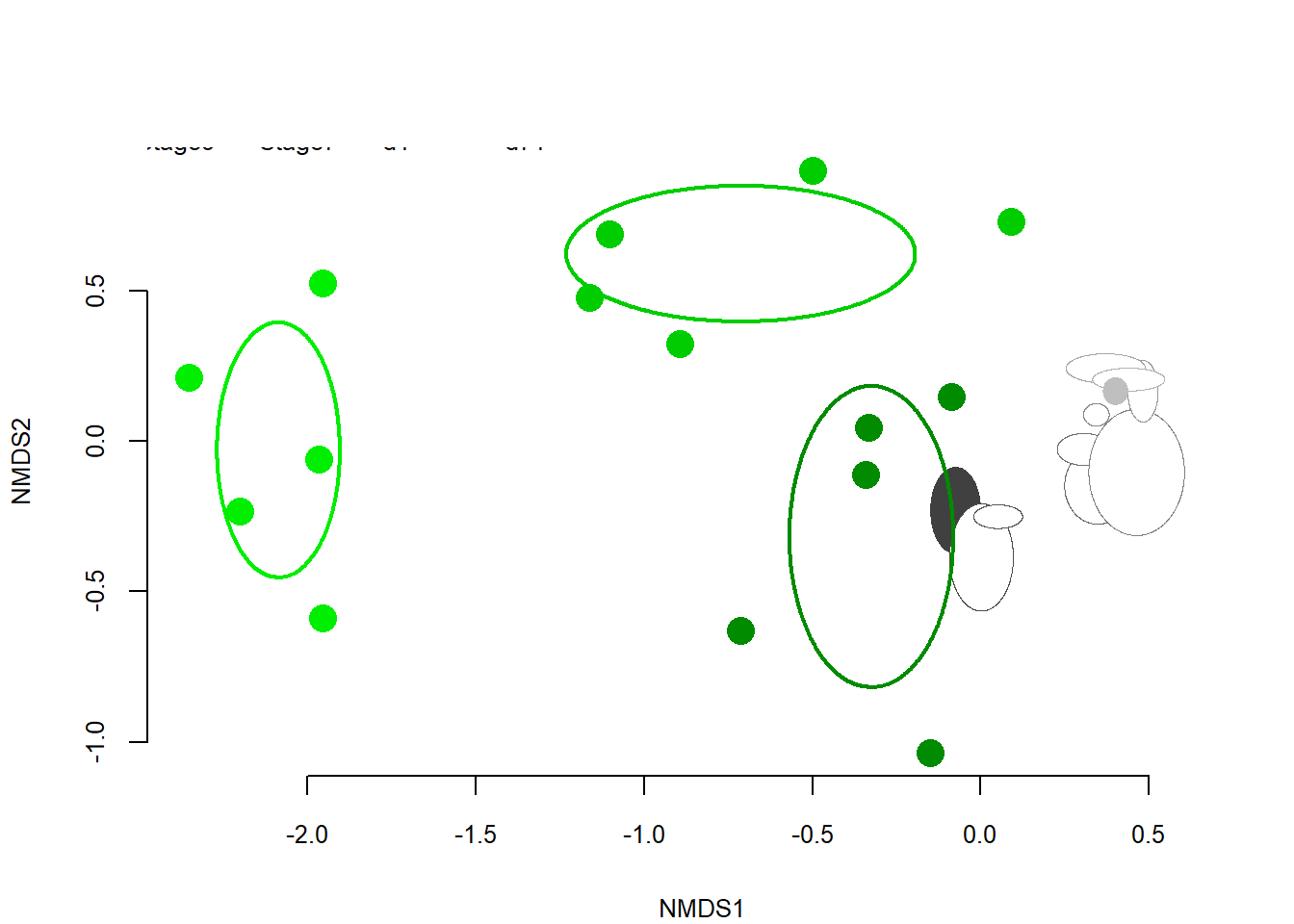# (0) Processing raw T-RFLP chromatogram files and create data matrix according to Abdo et al. (2006)

# Using the source code from Abdo et al. (2006). Statistical methods for characterizing diversity of microbial communities by analysis of terminal restriction fragment length polymorphisms of 16S rRNA genes. Environmental Microbiology, 8(5), 929–938. https://doi.org/10.1111/j.1462-2920.2005.00959.x
# The original source code was improved with easier data import and export parts.
# Use the raw Genemapper (v3.7) files. Use separate files for each enzyme! In our case it is Alu and Hin6I
# Example of the header and the first raws----
# Dye/Sample Peak   Sample File Name    Size    Height  Area    Data Point
# "Y,1" TA01_Alu_0.5 - 1-29-15-12-34 PM.fsa 49.9    234 1836    3308
# "Y,2" TA01_Alu_0.5 - 1-29-15-12-34 PM.fsa 52.46   57  541 3335
# "Y,3" TA01_Alu_0.5 - 1-29-15-12-34 PM.fsa 54.24   116 1140    3354

# You can have spaces in sample names.
# Check whether there is a tabulator after the 'Data point' column. If yes, then 'Number of columns' are 7, otherwise 6.

# Load source code for T-RFLP data processing----
source("Abdo-T-RFLP_core.r")

# Abdo_TRF.ftn("File name containing the raw chromatograms", "Enzyme name","Enzyme nave abbreviated", Number of coloumns usually 7, Multiplier for standard deviation usually 3, Bin,Length of sample name)
# For standard deviation and bin, please check Abdo et al. (2006)
# Use points for decimal points in chromatogram files!
# The resulted T-RFLP data matrix will be exported to the working directory as text file.

# Run Abdo code for first enzyme, which include data export, as well----
Abdo_TRF.ftn("L2012_Alu.txt","Alu","A",7, 4, 1, 6)
##                TA01        TA02        TA03 TA12       TA22        TA32
## A_49.67 0.004785662 0.005167502 0.004304676    0 0.00000000 0.004021874
## A_52.77 0.000000000 0.000000000 0.000000000    0 0.00000000 0.000000000
## A_54.71 0.000000000 0.000000000 0.000000000    0 0.01430305 0.000000000
## A_56.03 0.000000000 0.006680420 0.004386632    0 0.00000000 0.000000000
## A_57.09 0.000000000 0.000000000 0.000000000    0 0.00000000 0.000000000
## A_58.18 0.000000000 0.000000000 0.000000000    0 0.00000000 0.000000000
# If you would like to work with the resulted TRF_df data matrix further in R, then rename it
# paste("File name", 'Standard deviation', 'Bin',sep="_")

assign(paste("L2012_A", 4, 1,sep="_"),TRF_df)
head(L2012_A_4_1)[,1:6]
##                TA01        TA02        TA03 TA12       TA22        TA32
## A_49.67 0.004785662 0.005167502 0.004304676    0 0.00000000 0.004021874
## A_52.77 0.000000000 0.000000000 0.000000000    0 0.00000000 0.000000000
## A_54.71 0.000000000 0.000000000 0.000000000    0 0.01430305 0.000000000
## A_56.03 0.000000000 0.006680420 0.004386632    0 0.00000000 0.000000000
## A_57.09 0.000000000 0.000000000 0.000000000    0 0.00000000 0.000000000
## A_58.18 0.000000000 0.000000000 0.000000000    0 0.00000000 0.000000000
# Then do the same for second enzyme, which include data export, as well----
Abdo_TRF.ftn("L2012_Hin.txt","Hin","H",7, 4, 1, 6)
##                TA01        TA02        TA03 TA12 TA22 TA32
## H_49.57 0.005737052 0.004234920 0.004739789    0    0    0
## H_52.11 0.000000000 0.000000000 0.000000000    0    0    0
## H_54.23 0.000000000 0.000000000 0.000000000    0    0    0
## H_55.25 0.000000000 0.000000000 0.000000000    0    0    0
## H_56.73 0.006293512 0.004992253 0.007060669    0    0    0
## H_58.35 0.008325570 0.000000000 0.005224630    0    0    0
assign(paste("L2012_H", 4, 1,sep="_"),TRF_df)
head(L2012_H_4_1)[,1:6]
##                TA01        TA02        TA03 TA12 TA22 TA32
## H_49.57 0.005737052 0.004234920 0.004739789    0    0    0
## H_52.11 0.000000000 0.000000000 0.000000000    0    0    0
## H_54.23 0.000000000 0.000000000 0.000000000    0    0    0
## H_55.25 0.000000000 0.000000000 0.000000000    0    0    0
## H_56.73 0.006293512 0.004992253 0.007060669    0    0    0
## H_58.35 0.008325570 0.000000000 0.005224630    0    0    0
# The exported resulted alignment was compared to the raw chromatograms and corrected manually if needed in Excel. Then the data matrices for the two enzymes were combined, and this corrected data matrix was imported into R.

# (i) Comparing within and among block heterogeneity at selected sampling dates

# Calculate Bray-Curtis distances at day 1, 30 and 57. Then testing the differences among the two type of distances (within and among block) for the mentioned sampling dates with t-test.

# Read data matrix, which was manually corrected in Excel----
# Sample names are coded as following:
# e.g. TA01
# 'T' is the general code for this work,
# 'A' is the block name from 'A' to 'E'
# 0 is the number of sampling in weeks from 0 to 9 and 'L' as last
# 1 shows the parallel samples from one block.

# Create vector for sampling time
LWeek=substr(colnames(L2012),3,3)
LWeek=gsub("L",10,LWeek, fixed=TRUE)
LWeek=as.numeric(LWeek)
LWeek=rbind(LWeek)
# Create vector for block name
LBlock=substr(colnames(L2012),2,2)

# Put week number, block name and data matrix together
L2=rbind(LWeek[1:88],LBlock,L2012)
row.names(L2)[1:2]=c("Week","Block")

# Transpose data matrix and correct the type of values
L2=t(L2)
L2=as.data.frame(L2)
L2[,3:239] <- apply(L2[,3:239], 2, as.numeric)
L2[,1:2] <- apply(L2[,1:2], 2, as.character)

# Calculate Bray-Curtis distances within and among blocks on day 1, 30 and 57, that is Week 0, 4 and 8----

# Select weeks
WeekType=c(0,4,8)
# Get the name of unique blocks
BlockType=unique(L2[,2])
# Create empty objects
Dist_within_among=NULL

# library(vegan)

for (i in 1:length(WeekType)){
Week.i <- WeekType[i]
TRF.i <- L2[L2[,1] == Week.i, ]
Dist.i=vegdist(TRF.i[,3:239],method="bray")
DistM.i=as.matrix(Dist.i)
# Create all possible combinations for coloumn headers
xy <- t(combn(colnames(DistM.i), 2))
xy
# Recall matrix according to coloumn header combinations
DistList.i=data.frame(xy, dist=DistM.i[xy])
# DistList.i

# Re-arrange them to coloumns according to among and within distances
Dist_within.i=NULL
Dist_among.i=NULL
for (j in 1:length(DistList.i[,1])){
if(substr(DistList.i[j,1],2,2)==substr(DistList.i[j,2],2,2)){Dist_within.i=c(Dist_within.i,DistList.i[j,3])
}    else{Dist_among.i=c(Dist_among.i,DistList.i[j,3])}
}
Dist_within.i=c(Dist_within.i,rep(NA,105-length(Dist_within.i)))
Dist_among.i=c(Dist_among.i,rep(NA,105-length(Dist_among.i)))

Dist_within_among=cbind(Dist_within_among,Dist_within.i,Dist_among.i)

}

# Plot within and among block distances----
Plot_col=rep(c("white","gray"),3)
boxplot(Dist_within_among,col=Plot_col,xlab="",ylab="Bray-Curtis distances",font.lab=2,cex.lab=1.2)
title("Within and among block distances")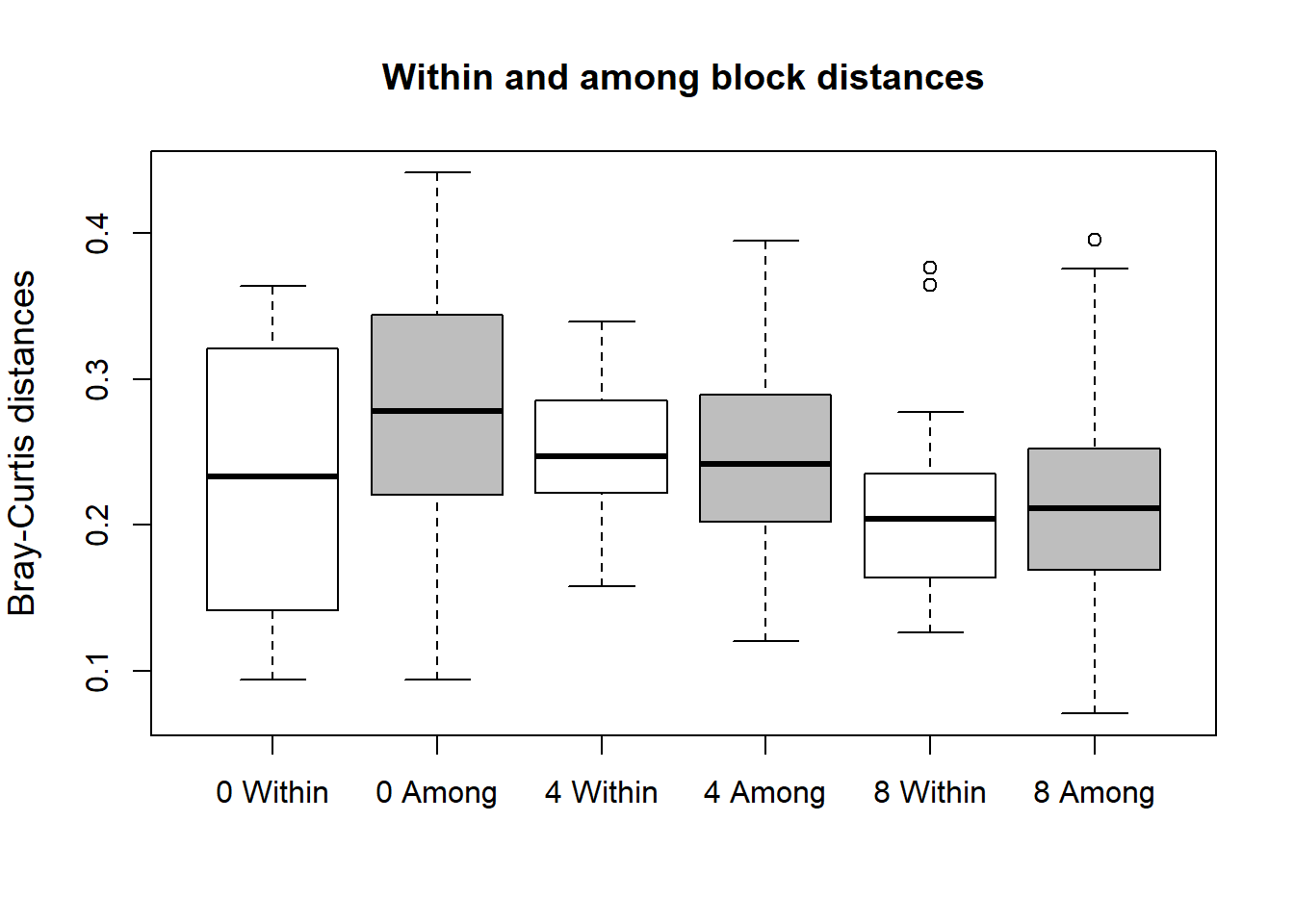# Export resulted Bray-Curtis distances----
write.table(Dist_within_among,file="Within and among block BC-distances week 0,4,8.txt",sep="\t",dec=".",row.names=T,col.names=T)

# Test normality within every type of distances
Norm_Shap_TavBK=apply(Dist_within_among,2,shapiro.test)

# T-tests comparing within and among block distances for Week 0, 4, 8----
# Use Monte Carlo resampling from Among-block values
Week_within_among_t_test=NULL
Within_number=c(1,3,5)
Among_number=Within_number+1
for (j in 1:length(Within_number)){
T_test=NULL
for (i in 1:1000){
Tt.i=t.test(sample(na.omit(Dist_within_among[,Within_number[j]]),13),sample(na.omit(Dist_within_among[,Among_number[j]]),13))
T_test=c(T_test,Tt.i$p.value) } Week_within_among_t_test=rbind(Week_within_among_t_test,c(median(T_test),mean(T_test))) } colnames(Week_within_among_t_test)=c("t_test p values Median_MC","t_test p values Mean_MC") row.names(Week_within_among_t_test)=c("day 1","day 30","day 57") Week_within_among_t_test ## t_test p values Median_MC t_test p values Mean_MC ## day 1 0.2644049 0.3199324 ## day 30 0.6648558 0.6225121 ## day 57 0.6488069 0.6263735 write.table(Week_within_among_t_test,"T-test comparing within and among block heterogeneity BC distances week 0,4,8.txt",sep="\t",dec=",",row.names=T) # Create data matrix for so-called relevant samples---- # None of the sampling dates showed significant differences (p>0.1) among the two type of distances. Hence, we decided to use only one parallel sample from each block, which were called relevant samples in the followings. # This relevant dataset contained 55 samples (11 sampling dates, one-one sample from the 5 mushroom blocks). row.names(L2) ##  "TA01" "TA02" "TA03" "TB01" "TB02" "TB03" "TC01" "TC02" "TC03" "TD01" ##  "TD02" "TE01" "TE02" "TE03" "TA12" "TB13" "TC12" "TD11" "TE12" "TA22" ##  "TB23" "TC23" "TD23" "TE22" "TA32" "TB32" "TC31" "TD33" "TE32" "TA41" ##  "TA42" "TA43" "TB41" "TB42" "TB43" "TC41" "TC42" "TC43" "TD42" "TD43" ##  "TE41" "TE42" "TE43" "TA53" "TB52" "TC52" "TD51" "TE52" "TA61" "TB62" ##  "TC63" "TD62" "TE62" "TA71" "TB71" "TC73" "TD72" "TE71" "TA81" "TA82" ##  "TA83" "TB81" "TB82" "TB83" "TC81" "TC82" "TC83" "TD81" "TD82" "TD83" ##  "TE81" "TE82" "TE83" "TA93" "TB92" "TC93" "TD93" "TE92" "TAL1" "TAL6" ##  "TBL3" "TBL5" "TCL3" "TCL5" "TDL2" "TDL4" "TEL2" "TEL5" Relevant_samples=c("x", 0, 0, "x", 0, 0, "x", 0, 0, "x", 0, "x", 0, 0, "x", "x", "x", "x", "x", "x", "x", "x", "x", "x", "x", "x", "x", "x", "x", "x", 0, 0, 0, "x", 0, "x", 0, 0, "x", 0, "x", 0, 0, "x", "x", "x", "x", "x", "x", "x", "x", "x", "x", "x", "x", "x", "x", "x", "x", 0, 0, "x", 0, 0, "x", 0, 0, "x", 0, 0, "x", 0, 0, "x", "x", "x", "x", "x", "x", 0, "x", 0, "x", 0, "x", 0, "x", 0) names(Relevant_samples)=row.names(L2) L2=cbind(Relevant_samples,L2) L2_r = L2[L2[,1] == "x", ] # Search and then remove those T-RFs, which has only 0 abundance in the relevant samples L2_r[56,] =c(NA,NA,NA,apply(L2_r[,4:240], 2, sum)) row.names(L2_r)="TRF_sum" L2_r_t=t(L2_r[,4:240]) L2_r_filter=t(L2_r_t[L2_r_t[,56] != 0,]) L2_r = cbind(L2_r[,2:3],L2_r_filter) L2_r=head(L2_r,-1) # Check the selection of relevant samples identical(L2_r$Block,rep(c("A","B","C","D","E"),11))
##  TRUE
identical(L2_r$Week,as.character(sort(rep(c(0:10),5)))) ##  TRUE # Export final data matrix for relevant samples---- write.table(L2_r,file="L2012_4_1_merg_final_relevant_samples.txt",row.names=T,col.names=T,dec=".",sep="\t") # (ii) Succession of the bacterial community was visualized with non-metric multidimensional scaling (NMDS) using Bray-Curtis distances (Fig1A) # Read final data matrix for relevant samples---- L2_r = read.table(file="L2012_4_1_merg_final_relevant_samples.txt",head=T,row.names = 1,sep="\t",dec=".") # Generate vectors for block names LBlock=substr(row.names(L2_r),2,2) LBlock_shape=LBlock for(i in 1:length(unique(LBlock_shape))){ LBlock_shape=gsub(as.character(unique(LBlock_shape)[i]),20+i,LBlock_shape, fixed=TRUE)} LBlock_shape_num=as.numeric(LBlock_shape) # Perform NMDS and calculate coordinates for weekly averages---- L_MDS<-metaMDS(L2_r[,3:222]) ## Wisconsin double standardization ## Run 0 stress 0.1293563 ## Run 1 stress 0.1300048 ## Run 2 stress 0.1685234 ## Run 3 stress 0.1656813 ## Run 4 stress 0.1452819 ## Run 5 stress 0.1437431 ## Run 6 stress 0.154736 ## Run 7 stress 0.1546163 ## Run 8 stress 0.1753878 ## Run 9 stress 0.1301767 ## Run 10 stress 0.1301841 ## Run 11 stress 0.1293563 ## ... Procrustes: rmse 8.246279e-06 max resid 4.775245e-05 ## ... Similar to previous best ## Run 12 stress 0.154736 ## Run 13 stress 0.1448867 ## Run 14 stress 0.1293182 ## ... New best solution ## ... Procrustes: rmse 0.00355169 max resid 0.02453633 ## Run 15 stress 0.1673904 ## Run 16 stress 0.1458151 ## Run 17 stress 0.1426769 ## Run 18 stress 0.1452819 ## Run 19 stress 0.1452715 ## Run 20 stress 0.1527372 ## *** No convergence -- monoMDS stopping criteria: ## 15: stress ratio > sratmax ## 5: scale factor of the gradient < sfgrmin L_NMDS_xy <- scores(L_MDS, scaling = 2) L_NMDS_mean=aggregate(L_NMDS_xy,list(group=L2_r$Week),mean)
L_NMDS_sd=aggregate(L_NMDS_xy,list(group=L2_r$Week),sd) # NMDS figure (Fig1A)---- par(mfrow=c(1,1),mar = c(4,4,1,1),oma=c(0,0,0,0)) # library(shape) # library(calibrate) plot.new() plot.window(xlim=range(L_NMDS_xy[,1]), ylim=range(L_NMDS_xy[,2]), asp = NA) # Ellipses mark the weekly mean ± SD area based on the 5 relevant samples NMDS coordinates' for (i in 1:length(L_NMDS_mean[,2])){ plotellipse(rx = L_NMDS_sd$NMDS1[i], ry = L_NMDS_sd$NMDS2[i], mid = c(L_NMDS_mean[i,2],L_NMDS_mean[i,3]),lcol=gray((L_NMDS_mean$group[i]*0.7)/11),col=gray(35:45/50)[i])}
# Small symbols denote samples from different mushroom blocks (A-E)
points(L_NMDS_xy,cex = 1, pch=LBlock_shape_num, col="black", bg=gray((L2_r$Week/10))) # Mark samples subjected to NGS with blue symbols NGS_sample=c("TA01","TA41","TA81","TB01","TB42","TB81") LBlock_shape_num_NGS=as.data.frame(LBlock_shape_num) row.names(LBlock_shape_num_NGS)=row.names(L2_r) points(L_NMDS_xy[NGS_sample,],cex = 1.5, pch=LBlock_shape_num_NGS[NGS_sample,], col="black", bg="blue") # Add legend legend("topright", LETTERS[1:5],pch = c(21:25), bg ="white",cex=0.8) # Add arrows point from week_n mean to week_n+1 mean Arrows(L_NMDS_mean[1:10,2], L_NMDS_mean[1:10,3],L_NMDS_mean[2:11,2], L_NMDS_mean[2:11,3],arr.type = "triangle",arr.length = 0.2) # Add the name of sampling days Sampling_days=c("d1","d8","d12","d22","d30","d36","d43","d50","d57","d64","d71") textxy(L_NMDS_mean[,2],L_NMDS_mean[,3],Sampling_days,cex=1,col="red") axis(1,cex.axis=0.8) axis(2,cex.axis=0.8) title(xlab="NMDS1",ylab="NMDS2",cex.lab=0.8)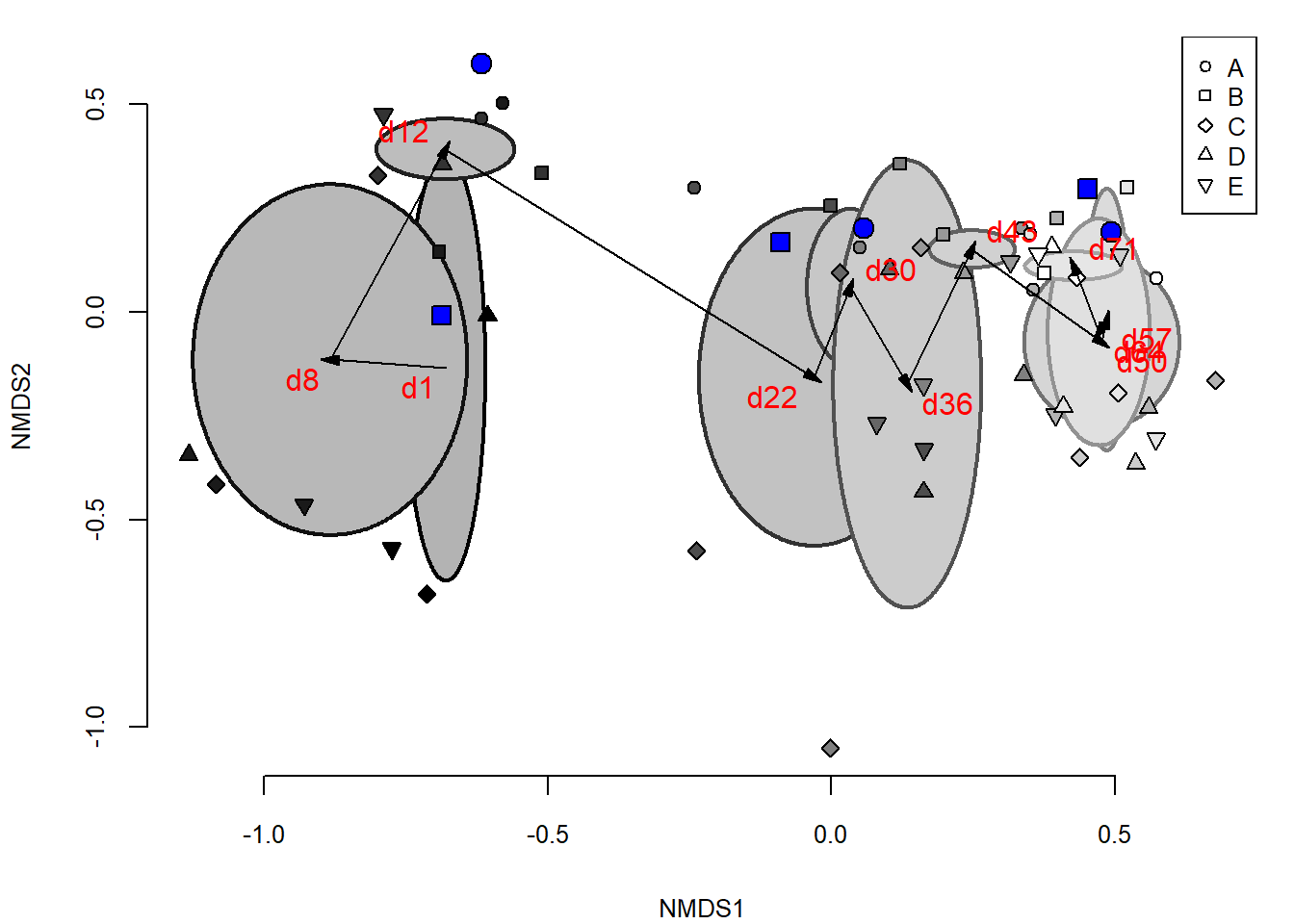# (iii) To test whether a priori grouping of samples according to sampling dates were separated significantly from each other Combined Cluster and Discriminant Analysis (CCDA) was applied (SFig1) # library(ccda) # Read final data matrix for relevant samples---- L2_r = read.table(file="L2012_4_1_merg_final_relevant_samples.txt",head=T,row.names = 1,sep="\t",dec=".") # Option for Bray-Curtis distance is added to raw CCDA code---- source("ccda.main.BC_Euclid.Ward.r") # CCDA using Bray-Curtis distance---- CCDA_Week_BC<-ccda.main_BC_Euclid(L2_r[,3:222], as.factor(L2_r$Week), 500, paste((unique(L2_r$Week)),sep = "",""), "proportions",return.RCDP=TRUE,Dist_method = "bray") ## ## Number of optimal groups: 5 ## ## Maximal difference between q95 and ratio 0.072 ## ## further investigation of the following 5 sub-groups recommended: [,1] ## 0 "sub-group 1" ## 1 "sub-group 2" ## 2 "sub-group 3" ## 3 "sub-group 4" ## 4 "sub-group 4" ## 5 "sub-group 4" ## 6 "sub-group 4" ## 7 "sub-group 5" ## 8 "sub-group 5" ## 9 "sub-group 5" ## 10 "sub-group 5" source("plotccda.cluster_group_names.r") plotccda.results(CCDA_Week_BC)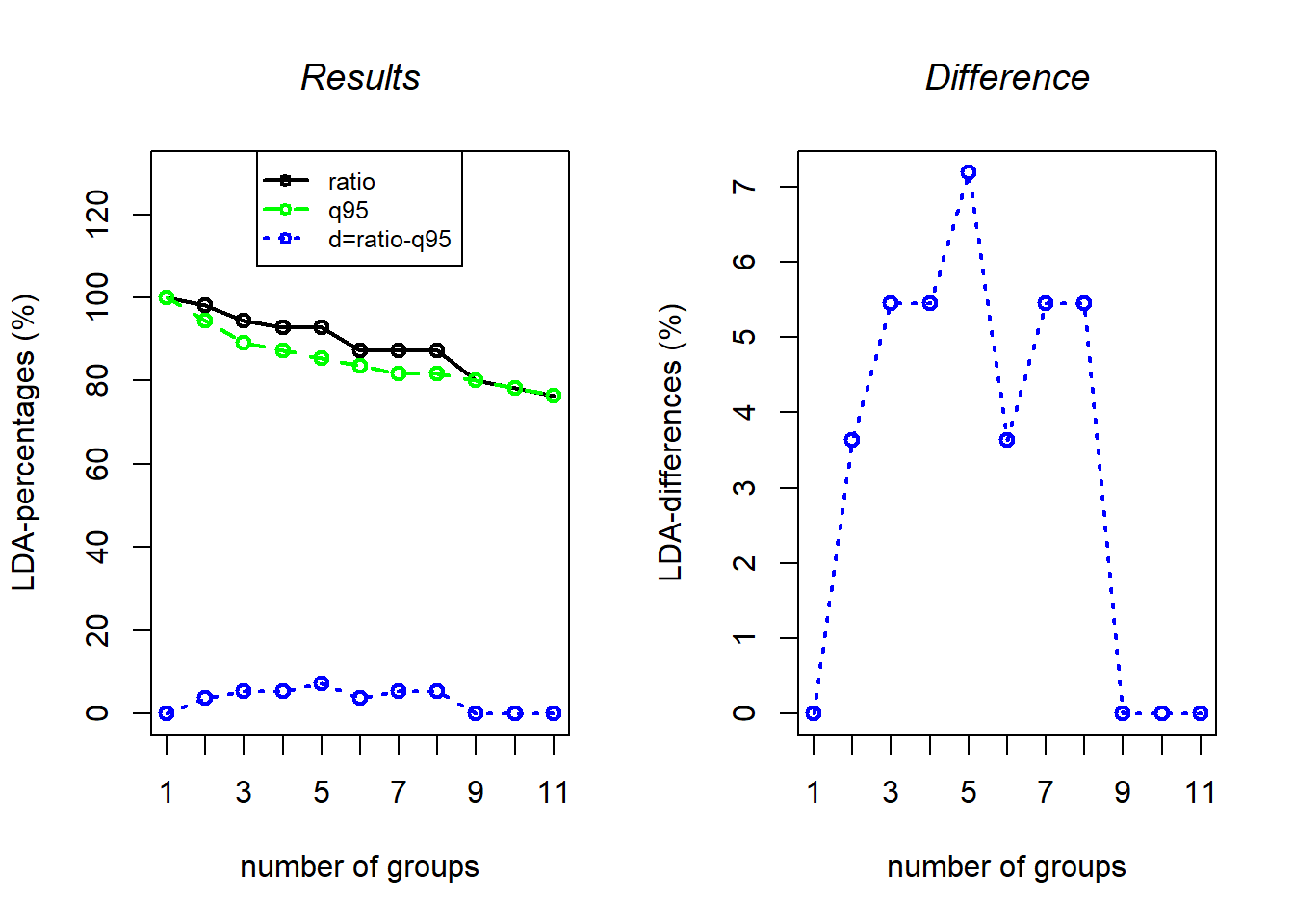Sampling_days=c("d1","d8","d12","d22","d30","d36","d43","d50","d57","d64","d71") plotccda.cluster_group_names(CCDA_Week_BC,Sampling_days,"Bray-Curtis")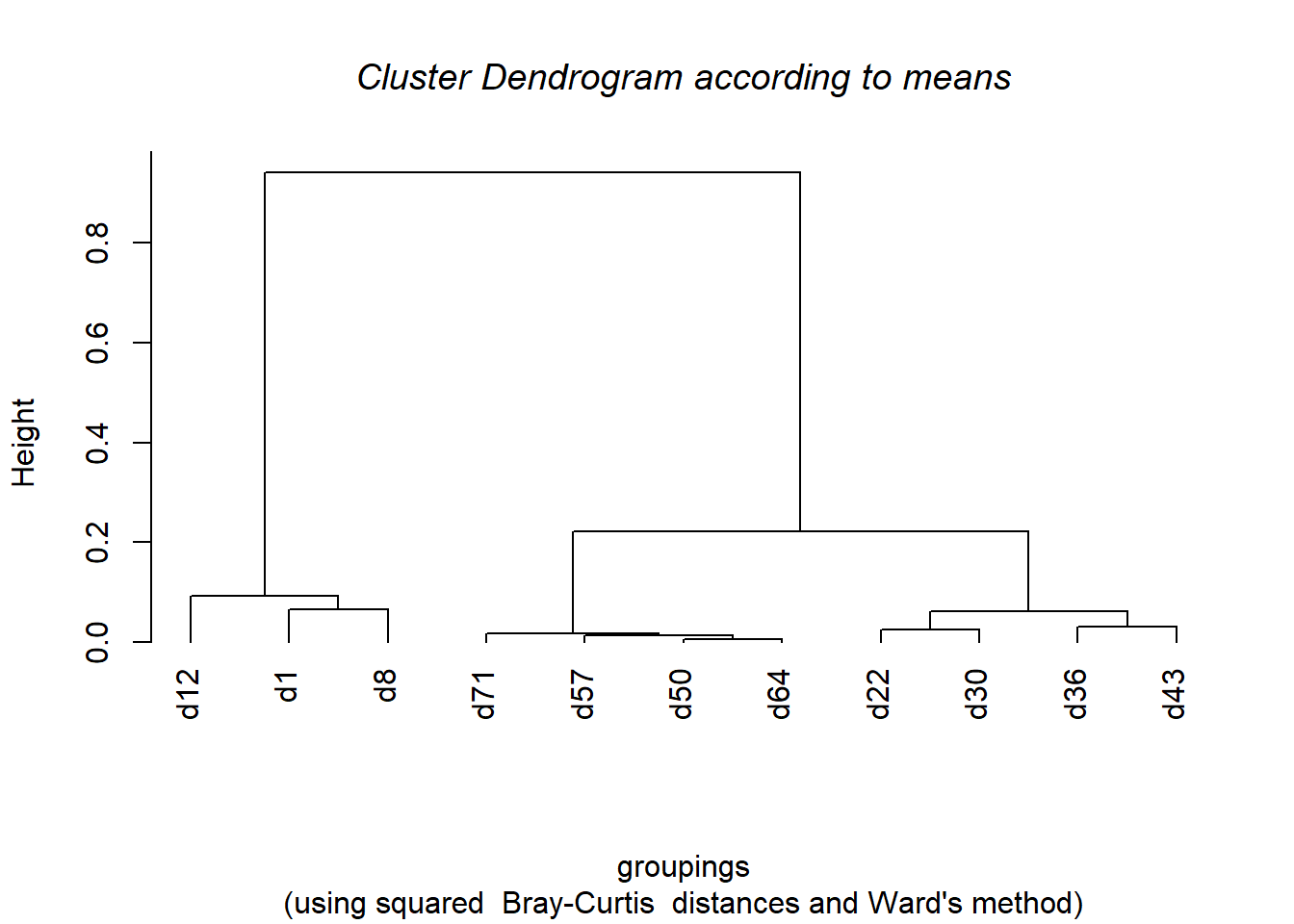## ## Call: ## hclust(d = mean_mtx_bc * mean_mtx_bc, method = mth) ## ## Cluster method : ward.D ## Distance : bray ## Number of objects: 11 CCDA_Week_BC$sub_groups
##    further investigation of the following  5 sub-groups  recommended:
## 0  "sub-group 1"
## 1  "sub-group 2"
## 2  "sub-group 3"
## 3  "sub-group 4"
## 4  "sub-group 4"
## 5  "sub-group 4"
## 6  "sub-group 4"
## 7  "sub-group 5"
## 8  "sub-group 5"
## 9  "sub-group 5"
## 10 "sub-group 5"

# (iv) Shannon diversity indices were calculated (SFig2)

# library(PMCMR)
# library(multcompView)
# Read final data matrix for relevant samples----
# Calculate Shannon diversity----
ShanL=diversity(L2_r[,3:222],index = "shannon")

# Formatting data for further statistical tests
L_Div=as.data.frame(cbind(ShanL,L2_r$Week)) colnames(L_Div)="Group" L_Div$Group=as.factor(L_Div$Group) THK_tx=L_Div$Group

# Testing significant differences among groups with Kruskal-Wallis test----
(KW.p<-signif(kruskal.test(ShanL~THK_tx, data=L_Div)$p.value,3)) ##  0.00351 # Kruskal-Neményi post-hoc test (PMCMR csomag) for finding significantly different groups---- (KN.m=posthoc.kruskal.nemenyi.test(ShanL~THK_tx, data=L_Div, dist="Tukey")$p.value)
##             0         1          2         3         4         5         6
## 1  0.42721785        NA         NA        NA        NA        NA        NA
## 2  0.99999995 0.6396117         NA        NA        NA        NA        NA
## 3  0.94345025 0.9984467 0.98935509        NA        NA        NA        NA
## 4  0.99559126 0.9686299 0.99978191 0.9999986        NA        NA        NA
## 5  0.45478566 1.0000000 0.66737353 0.9989487 0.9747805        NA        NA
## 6  0.74645149 0.9999967 0.89917429 0.9999979 0.9989487 0.9999986        NA
## 7  0.02857700 0.9919374 0.07130452 0.6673735 0.3739794 0.9893551 0.9065627
## 8  0.13530698 0.9999786 0.26668451 0.9381625 0.7464515 0.9999592 0.9955913
## 9  0.03690287 0.9955913 0.08910069 0.7209341 0.4272179 0.9939880 0.9325393
## 10 0.27761860 1.0000000 0.46875737 0.9893551 0.9065627 1.0000000 0.9998311
##            7         8         9
## 1         NA        NA        NA
## 2         NA        NA        NA
## 3         NA        NA        NA
## 4         NA        NA        NA
## 5         NA        NA        NA
## 6         NA        NA        NA
## 7         NA        NA        NA
## 8  0.9999848        NA        NA
## 9  1.0000000 0.9999967        NA
## 10 0.9989487 0.9999999 0.9995532
KN.out=posthoc.kruskal.nemenyi.test(ShanL~THK_tx, data=L_Div, dist="Tukey")
KN.out.p=get.pvalues(KN.out)
(KN.letter=multcompLetters(KN.out.p))
##    0    1    2    3    4    5    6    7    8    9   10
##  "a" "ab" "ab" "ab" "ab" "ab" "ab"  "b" "ab"  "b" "ab"
# Plotting Shannon diversity----
par(mar=c(3,3,3,1))
Sampling_days=c("d1","d8","d12","d22","d30","d36","d43","d50","d57","d64","d71")
boxplot(ShanL~THK_tx, data=L_Div,xlab="",ylab="",names=Sampling_days,srt=90, col=gray((0:10)/10),cex.lab=1.3,outline=F,las=1)
axis(3,at=1:11,labels=KN.letter$Letters,font = 1,las=1)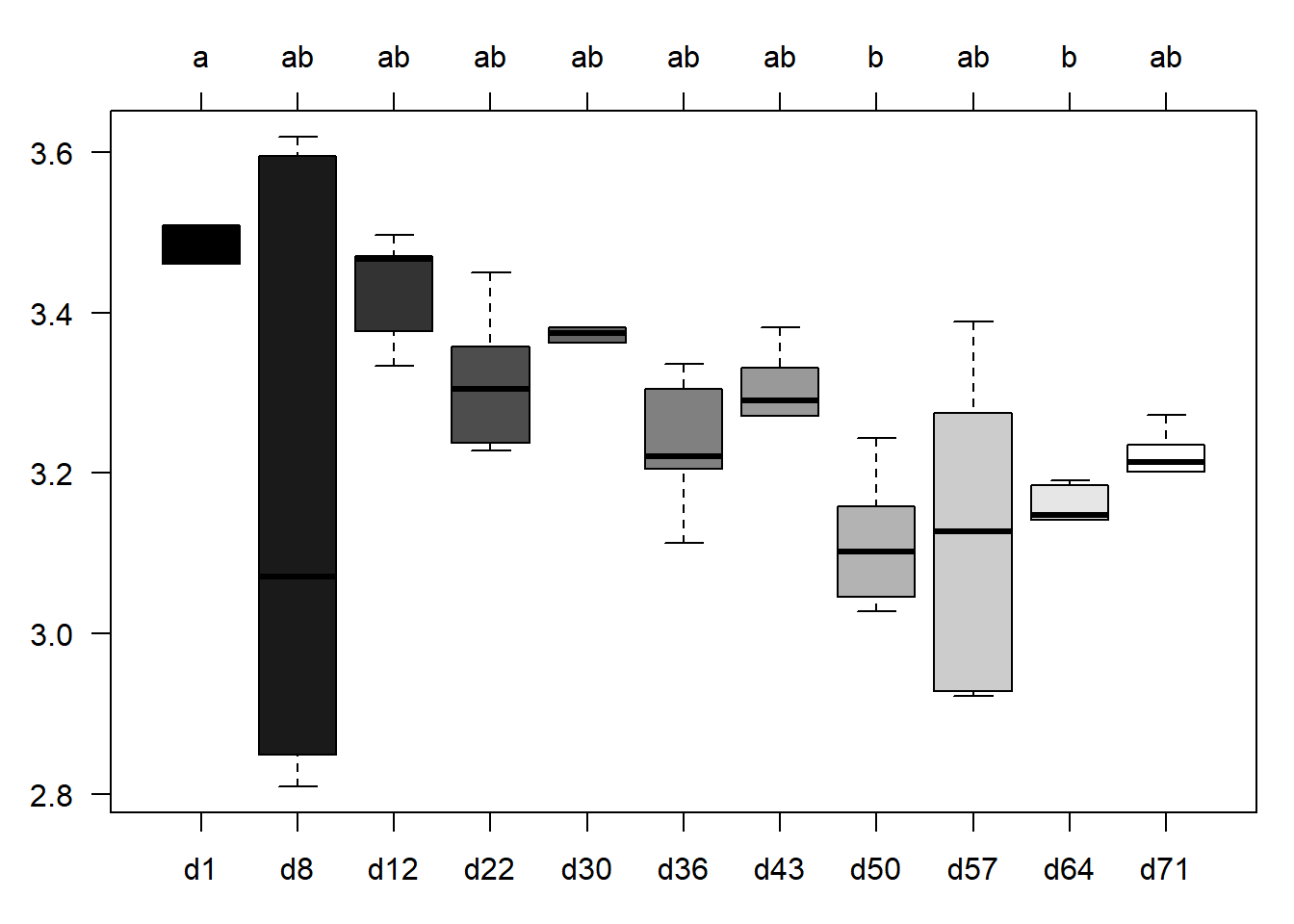# (v) Spatial and temporal changes in community composition were calculated (Fig1D) ## Distances among weeks: calculations # Read final data matrix for relevant samples and selected samples from P. ostreatus substrate preparation production series---- L_TS2012_r = read.table(file="L2012_TS_relev Abdo 4_1 merge final.txt",head=T,row.names = 1,sep="\t",dec=".") Week_among=c("S13","S37","1-8","8-12","12-22","22-30","30-36","36-43","43-50","50-57","57-64","64-71") Week_among_KN=c("S13","S37","1_8","8_12","12_22","22_30","30_36","36_43","43_50","50_57","57_64","64_71") Week_among_figure=c("S1-3","S3-7","d1-8","d8-12","d12-22","d22-30","d30-36","d36-43","d43-50","d50-57","d57-64","d64-71") Week_2012_relev=c("S01","S03","S07","d01","d08","d12","d22","d30","d36","d43","d50","d57","d64","d71") Week_2012_relev_fig=c("S01","S03","S07","d1","d8","d12","d22","d30","d36","d43","d50","d57","d64","d71") WeekType_r=unique(L_TS2012_r[,1]) BlockType_r=unique(L_TS2012_r[,2]) Dist_week_among=NULL Col_header_r=NULL Dist_week_among_same_block=NULL # Bray-Curtis distances among samples from week i. and i+1. for (i in 1:(length(WeekType_r)-1)){ Week_r.i <- WeekType_r[i] TRF.i <- rbind(L_TS2012_r[L_TS2012_r[,1] == Week_r.i, ],L_TS2012_r[L_TS2012_r[,1] == (as.numeric(Week_r.i)+1), ]) Dist_r.i=vegdist(TRF.i[,3:320],method="bray") DistM_r.i=as.matrix(Dist_r.i) # Create all possible combinations for coloumn headers xy <- t(combn(colnames(DistM_r.i), 2)) xy # Recall matrix according to coloumn headers DistList_r.i=data.frame(xy, dist=DistM_r.i[xy]) # Select only distances among weeks Dist_among_r.i=NULL Dist_within_r.i=NULL Dist_among_same_block.i=NULL for (j in 1:length(DistList_r.i[,1])){ if(substr(DistList_r.i[j,1],3,3)!=substr(DistList_r.i[j,2],3,3)){Dist_among_r.i=c(Dist_among_r.i,DistList_r.i[j,3])} # Remove distances among the same blocks week i. and week i+1. values if(substr(DistList_r.i[j,1],2,2)==substr(DistList_r.i[j,2],2,2)){Dist_among_same_block.i=c(Dist_among_same_block.i,DistList_r.i[j,3])} } Col_header_among_r=paste(Week_r.i,"-",(as.numeric(Week_r.i)+1)) Col_header_r=c(Col_header_r,Col_header_among_r) Dist_week_among=cbind(Dist_week_among,Dist_among_r.i) Dist_week_among_same_block=cbind(Dist_week_among_same_block,Dist_among_same_block.i) } # Distances among Stage7 (S7) and day1 is not interesting, it can be removed! Dist_week_among=cbind(Dist_week_among[,1:2],Dist_week_among[,4:13]) colnames(Dist_week_among)=Week_among_KN boxplot(Dist_week_among,col=c("green","greenyellow",gray((0:9)/9)),xlab="",ylab="",names=Week_among_figure,font.axis=2,cex.axis=0.7)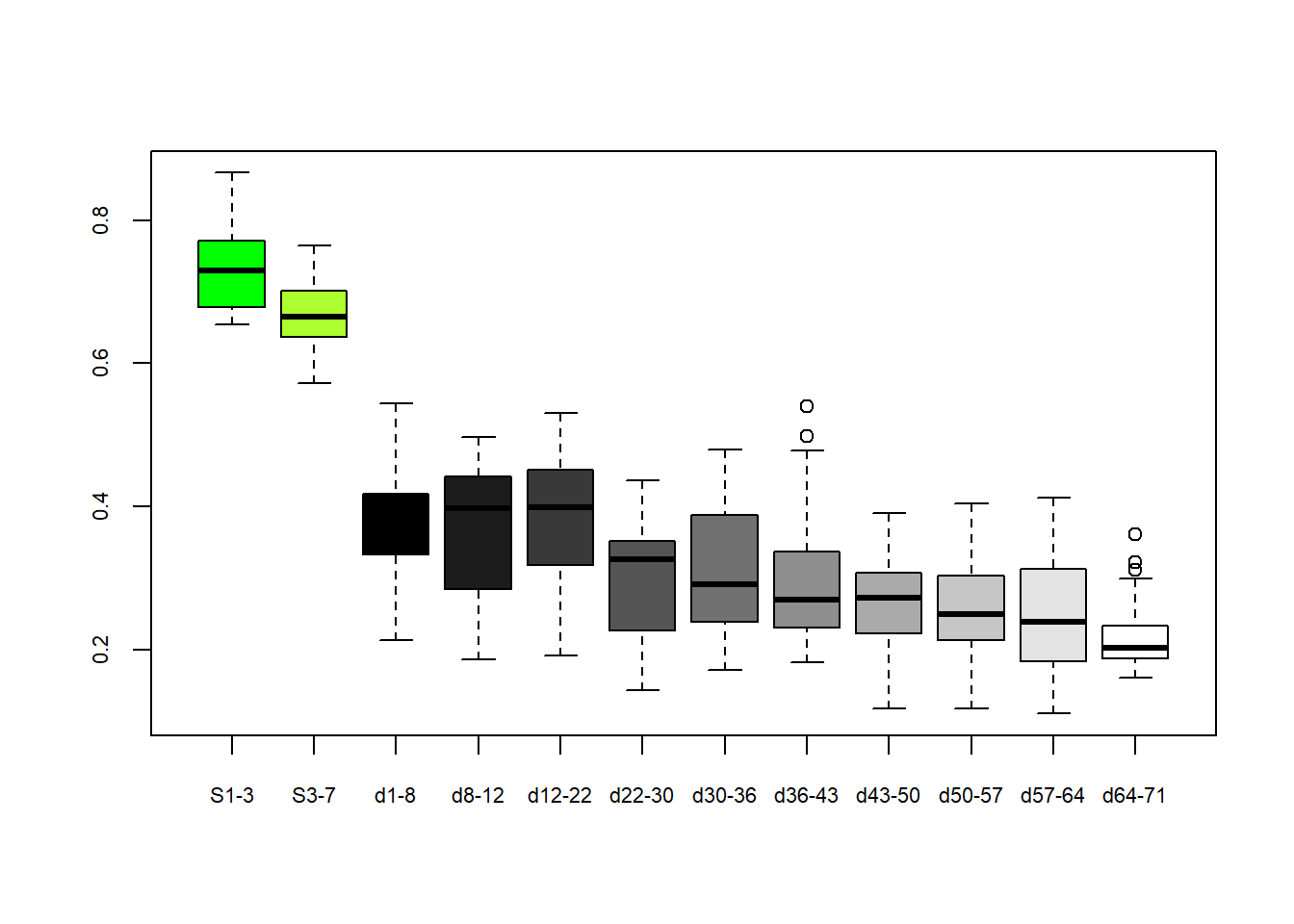## Distances among weeks: statistics List_Dist_week_among_r=melt(Dist_week_among, # ID variables - all the variables to keep but not split apart on # id.vars=c("subject", "sex"), # The source columns measure.vars=Week_among_KN, # Name of the destination column that will identify the original # column that the measurement came from variable.name="Group", value.name="Distance") List_Dist_week_among_r=List_Dist_week_among_r[,2:3] colnames(List_Dist_week_among_r)="Group" THK_tx=List_Dist_week_among_r$Group

# Checking homogeneity of variances: Bartlett and Cochran-tests----
# Bartlett test
(Ba.p<-signif(bartlett.test(Distance~THK_tx, data=List_Dist_week_among_r)$p.value,3)) ##  0.00704 #Cochran test: in case of inhomogeneous variance, who is the outlier THK_amod <- aov(Distance~THK_tx, data=List_Dist_week_among_r) Coh=C.test(THK_amod) (Ctp<-signif(C.test(THK_amod)$p.value,3))
##  0.236
# Kruskal-Wallis test----
(KW.p<-signif(kruskal.test(Distance~THK_tx, data=List_Dist_week_among_r)$p.value,3)) ##  1.13e-31 # Kruskal-Neményi post-hoc test (PMCMR csomag) for finding significantly different groups---- KN.m=posthoc.kruskal.nemenyi.test(Distance~THK_tx, data=List_Dist_week_among_r, dist="Tukey")$p.value
KN.out=posthoc.kruskal.nemenyi.test(Distance~THK_tx, data=List_Dist_week_among_r, dist="Tukey")
KN.out.p=get.pvalues(KN.out)
(KN.letter_among_week=multcompLetters(KN.out.p))
##   S13   S37   1_8  8_12 12_22 22_30 30_36 36_43 43_50 50_57 57_64 64_71
##   "a"   "a"   "b"  "bc"   "b" "bcd" "bcd" "bcd" "bcd"  "cd"   "d"   "d"
# Boxplot among weeks distances with statitics----

par(mar=c(2,4,2,2))
boxplot(Dist_week_among,col=c("green","greenyellow",gray((0:9)/9)),xlab="",ylab="Bray-Curtis distances",names=Week_among_figure,font.axis=2,cex.axis=0.8)
axis(3,at=1:12,labels=KN.letter_among_week$Letters,font = 1,cex=0.8)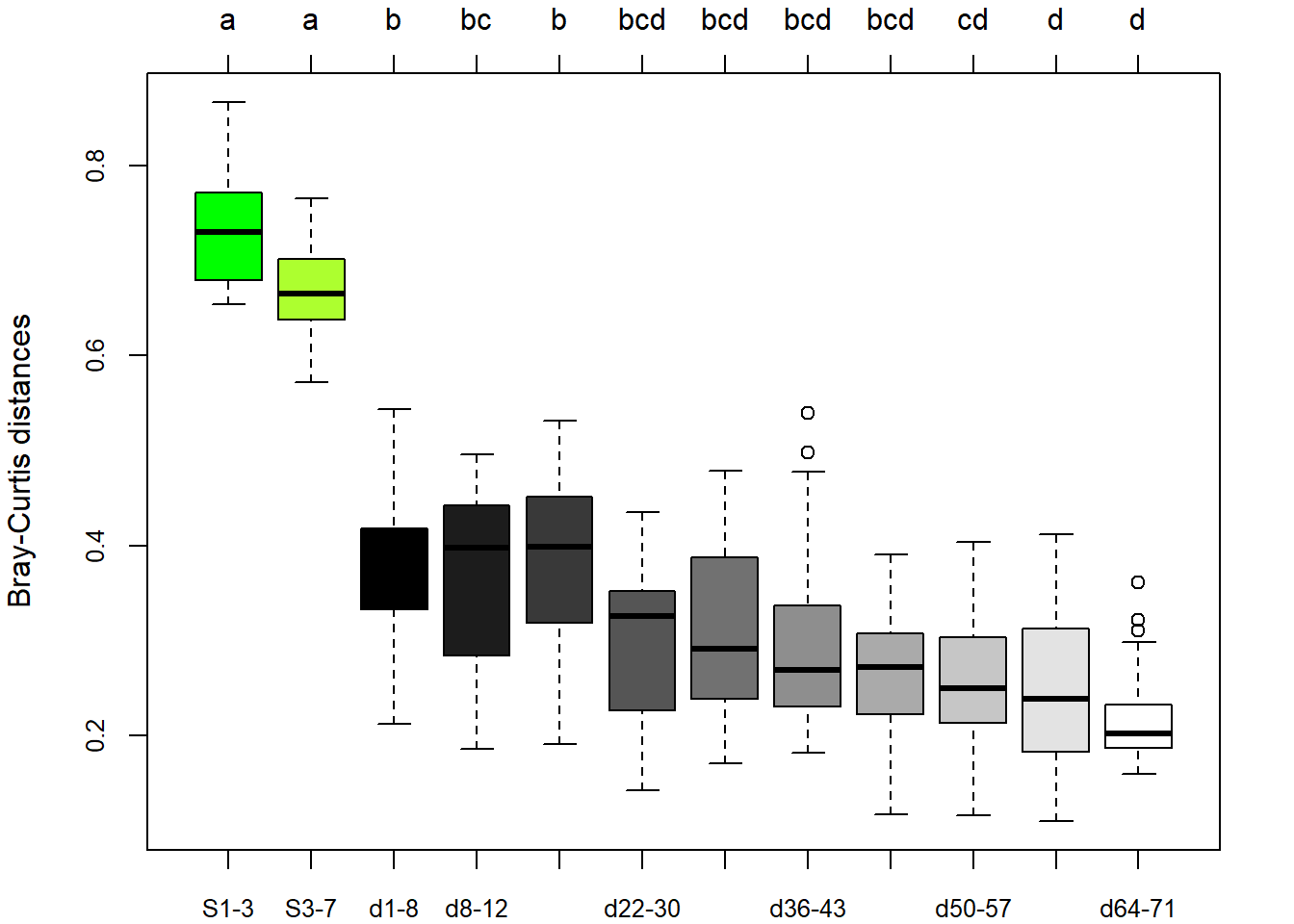## Distances within weeks: calculations WeekType=unique(L_TS2012_r[,1]) BlockType=unique(L_TS2012_r[,2]) Dist_among_r=NULL Col_header_r=NULL for (i in 1:length(WeekType)){ Week_r.i <- WeekType[i] TRF.i <- L_TS2012_r[L_TS2012_r[,1] == Week_r.i, ] Dist_r.i=vegdist(TRF.i[,3:222],method="bray") DistM_r.i=as.matrix(Dist_r.i) # Create all possible combinations for coloumn headers xy <- t(combn(colnames(DistM_r.i), 2)) xy # Recall matrix according to coloumn header combinations DistList_r.i=data.frame(xy, dist=DistM_r.i[xy]) # DistList_r.i # Re-arrenge them to coloumns according to among and within blocks Dist_within_r.i=NULL Dist_among_r.i=NULL for (j in 1:length(DistList_r.i[,1])){ if(substr(DistList_r.i[j,1],2,2)==substr(DistList_r.i[j,2],2,2)){Dist_within_r.i=c(Dist_within_r.i,DistList_r.i[j,3]) } else{Dist_among_r.i=c(Dist_among_r.i,DistList_r.i[j,3])} } Col_header_among_r=paste(Week_r.i) Col_header_r=c(Col_header_r,Col_header_among_r) # Combine distances within weeks, which are among samples form different blocks Dist_among_r=cbind(Dist_among_r,Dist_among_r.i) } colnames(Dist_among_r)=Week_2012_relev ## Distances within weeks: statistics Dist_List_week_within_r=melt(Dist_among_r, # ID variables - all the variables to keep but not split apart on # id.vars=c("subject", "sex"), # The source columns measure.vars=Week_2012_relev, # Name of the destination column that will identify the original # column that the measurement came from variable.name="Group", value.name="Distance") Dist_List_week_within_r=Dist_List_week_within_r[,2:3] colnames(Dist_List_week_within_r)="Group" THK_tx=Dist_List_week_within_r$Group

# Checking homogeneity of variances: Bartlett and Cochran-tests----
# Bartlett test
(Ba.p<-signif(bartlett.test(Distance~THK_tx, data=Dist_List_week_within_r)$p.value,3)) ##  0.000178 #Cochran test: in case of inhomogeneous variance, who is the outlier THK_amod <- aov(Distance~THK_tx, data=Dist_List_week_within_r) Coh=C.test(THK_amod) (Ctp<-signif(C.test(THK_amod)$p.value,3))
##  0.0235
(Ctalt<-substr(C.test(THK_amod)$alternative,7,9)) ##  "d08" # Kruskal-Wallis test---- (KW.p<-signif(kruskal.test(Distance~THK_tx, data=Dist_List_week_within_r)$p.value,3))
##  4.46e-12
# Kruskal-Neményi post-hoc test (PMCMR csomag) for finding significantly different groups----
KN.m=posthoc.kruskal.nemenyi.test(Distance~THK_tx, data=Dist_List_week_within_r, dist="Tukey")$p.value KN.out=posthoc.kruskal.nemenyi.test(Distance~THK_tx, data=Dist_List_week_within_r, dist="Tukey") KN.out.p=get.pvalues(KN.out) (KN.letter_within_week=multcompLetters(KN.out.p)) ## S01 S03 S07 d01 d08 d12 d22 d30 d36 d43 d50 ## "a" "a" "ab" "abcd" "abc" "bcd" "abcd" "bcd" "abcd" "cd" "bcd" ## d57 d64 d71 ## "abcd" "bcd" "d" # Boxplot within weeks distances with statitics---- par(mar=c(2,4,2,2)) boxplot(Dist_among_r,col=c("green2","green3","green4",gray((0:10)/10)),xlab="",ylab="Bray-Curtis distances",names=Week_2012_relev_fig,font.lab=2,cex.axis=0.8) axis(3,at=1:14,labels=KN.letter_within_week$Letters,font = 1,cex=0.8)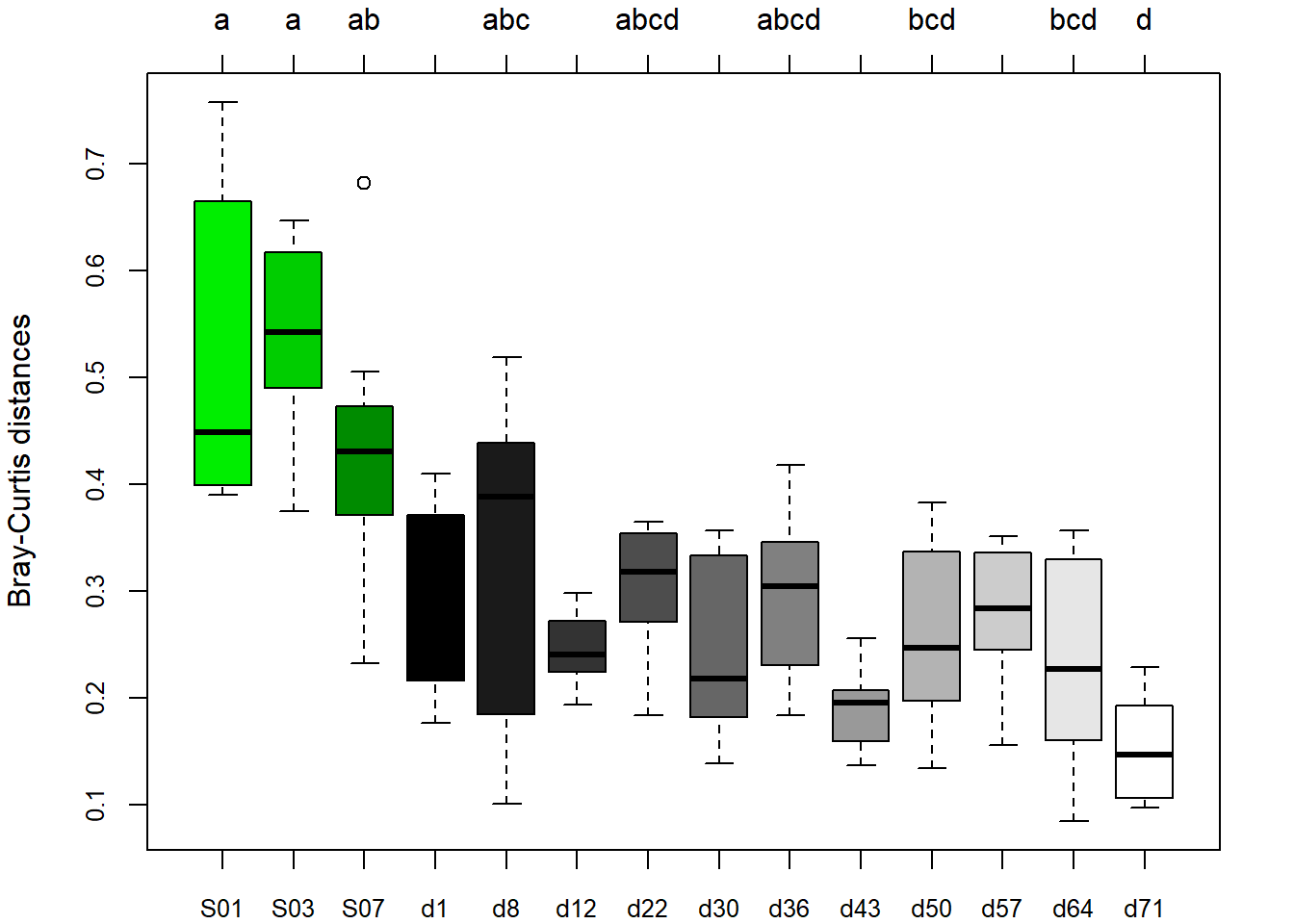## Spatial (cultivation block level) and temporal changes in community composition based on Bray-Curtis distances: Fig1D

# Combining the results from within and among week Bray-Curtis distances into one figure
AA=rbind(Dist_among_r,matrix(rep(rep(NA,15),14),ncol=14,nrow=15))
BB=Dist_week_among
AB=rbind(c(1,3,5,6,8,10,12,14,16,18,20,22,24,26,2,4,7,9,11,13,15,17,19,21,23,25),cbind(AA,BB))
Order_graphic=c(1,3,5,6,8,10,12,14,16,18,20,22,24,26,2,4,7,9,11,13,15,17,19,21,23,25)
AB=AB[,order(AB[1,],decreasing=F)]
Week_within_among_figure=c("S1",NA,"S3",NA,"S7","d1",NA,"d8",NA,"d12",NA,"d22",NA,"d30",NA,"d36",NA,"d43",NA,"d50",NA,"d57",NA,"d64",NA,"d71")

Col_Fig1D=c("green2","green3","green4",gray((0:10)/10),rep("NA",12))
Col_Fig1D=as.data.frame(cbind(Order_graphic,Col_Fig1D))
Col_Fig1D$Order_graphic=as.numeric(as.character(Col_Fig1D$Order_graphic))
Col_Fig1D=Col_Fig1D[order(Col_Fig1D[,1],decreasing=F),]
Col_Fig1D$Col_Fig1D=as.character(Col_Fig1D$Col_Fig1D)

PostHoc_within_week=KN.letter_within_week$Letters PostHoc_within_week=c(PostHoc_within_week,rep("",12)) PostHoc_within_week=as.data.frame(cbind(Order_graphic,PostHoc_within_week)) PostHoc_within_week$Order_graphic=as.numeric(as.character(PostHoc_within_week$Order_graphic)) PostHoc_within_week=PostHoc_within_week[order(PostHoc_within_week[,1],decreasing=F),] PostHoc_within_week$PostHoc_within_week=as.character(PostHoc_within_week$PostHoc_within_week) PostHoc_among_week=KN.letter_among_week$Letters
PostHoc_among_week=c(rep("",14),PostHoc_among_week)
PostHoc_among_week=as.data.frame(cbind(Order_graphic,PostHoc_among_week))
PostHoc_among_week$Order_graphic=as.numeric(as.character(PostHoc_among_week$Order_graphic))
PostHoc_among_week=PostHoc_among_week[order(PostHoc_among_week[,1],decreasing=F),]
PostHoc_among_week$PostHoc_among_week=as.character(PostHoc_among_week$PostHoc_among_week)

library(extrafont)
windowsFonts(
WA=windowsFont("Cabin Sketch"),
WB=windowsFont("Bookman Old Style"),
WC=windowsFont("Comic Sans MS"),
)

Outline_boxes=c(1,3,1,3,1,1,3,1,3,1,3,1,3,1,3,1,3,1,3,1,3,1,3,1,3,1)
par(mar=c(2,4,1,1))
boxplot(AB[-1,],col=Col_Fig1D[,2],xlab="",ylab="Bray-Curtis distances",names=Week_within_among_figure,font.lab=2,cex.axis=0.9,lty=Outline_boxes,ylim=c(0,0.9))
axis(3,at=1:26,labels=PostHoc_within_week$PostHoc_within_week,font = 1,cex.axis=0.8,line = -3.5,lwd = 0) axis(3,at=1:26,labels=PostHoc_among_week$PostHoc_among_week,font = 3,cex.axis=0.8,line = -15.5,lwd = 0,family="WA")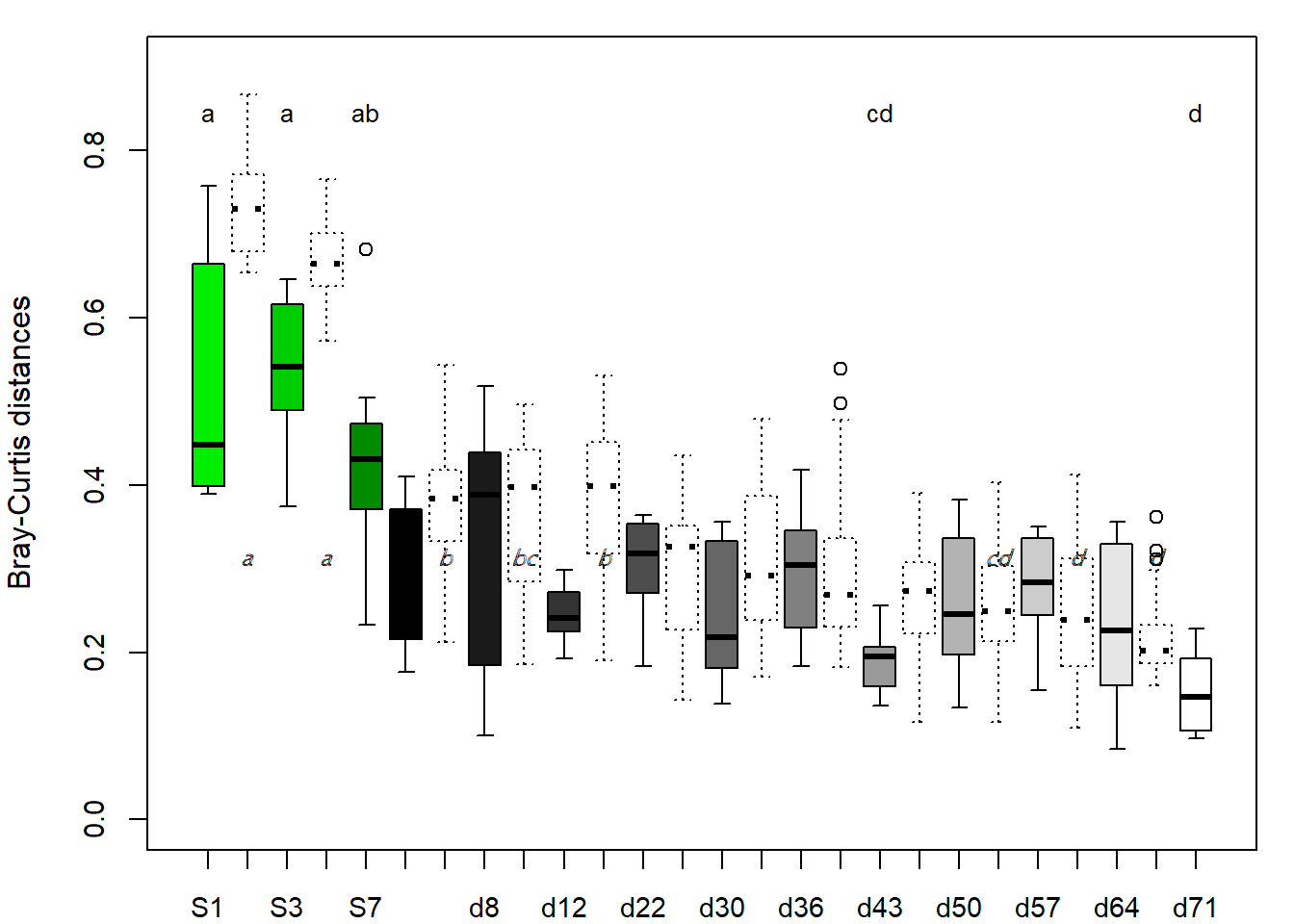# (vi) To test the effect of oyster mushroom lignocellulose-degrading enzymes on bacterial community composition, enzymatic activities were fitted as vectors with ‘envfit’ function onto the NMDS ordination: Fig1B

# Read enzymatic activity values----
# Selection of relevant samples
E2_r=E2[row.names(L2_r),]
# selfcheck
identical(row.names(L2_r),row.names(E2_r))
##  TRUE
# NMDS again for T-RFLP data of relevant samples----
# Perform NMDS and calculate coordinates for weekly averages
L_NMDS_E<-metaMDS(L2_r[,3:222])
## Wisconsin double standardization
## Run 0 stress 0.1293563
## Run 1 stress 0.1549624
## Run 2 stress 0.1551221
## Run 3 stress 0.1448717
## Run 4 stress 0.1537951
## Run 5 stress 0.1536416
## Run 6 stress 0.1293847
## ... Procrustes: rmse 0.004138285  max resid 0.02512868
## Run 7 stress 0.1293631
## ... Procrustes: rmse 0.001797602  max resid 0.01225962
## Run 8 stress 0.1301764
## Run 9 stress 0.1547288
## Run 10 stress 0.1452122
## Run 11 stress 0.1685235
## Run 12 stress 0.1301842
## Run 13 stress 0.1669224
## Run 14 stress 0.1568787
## Run 15 stress 0.1293631
## ... Procrustes: rmse 0.001814614  max resid 0.01237637
## Run 16 stress 0.1568324
## Run 17 stress 0.145306
## Run 18 stress 0.1547289
## Run 19 stress 0.1686723
## Run 20 stress 0.1293631
## ... Procrustes: rmse 0.001816072  max resid 0.01238616
## *** No convergence -- monoMDS stopping criteria:
##     17: stress ratio > sratmax
##      3: scale factor of the gradient < sfgrmin
L_NMDS_E_xy <- scores(L_NMDS_E, scaling = 2)
L_NMDS_E_mean=aggregate(L_NMDS_E_xy,list(group=L2_r$Week),mean) L_NMDS_E_sd=aggregate(L_NMDS_E_xy,list(group=L2_r$Week),sd)

# Envfit----
ef <- envfit(L_NMDS_E, E2_r[,3:10], permu = 10000)
ef
##
## ***VECTORS
##
##         NMDS1    NMDS2     r2    Pr(>r)
## Lacc -0.96702  0.25469 0.3820 9.999e-05 ***
## MnP   0.99997  0.00834 0.1860  0.005199 **
## Cbh   0.84243 -0.53881 0.2406  0.001100 **
## BglL  0.85844 -0.51291 0.1332  0.025297 *
## Exox  0.80319  0.59573 0.0231  0.533347
## Chit  0.99958  0.02898 0.2021  0.002900 **
## Cell  0.99917 -0.04083 0.0562  0.213779
## Xyl   0.97913  0.20323 0.0744  0.125287
## ---
## Signif. codes:  0 '***' 0.001 '**' 0.01 '*' 0.05 '.' 0.1 ' ' 1
## Permutation: free
## Number of permutations: 10000
# Final figure: NMDS and envfit----
par(mfrow=c(1,1),mar = c(4,4,1,1),oma=c(0,0,0,0))

plot.new()
plot.window(xlim=range(L_NMDS_E_xy[,1]),
ylim=range(L_NMDS_E_xy[,2]), asp = NA)
for (i in 1:length(L_NMDS_E_mean[,2])){
plotellipse(rx = L_NMDS_E_sd$NMDS1[i], ry = L_NMDS_E_sd$NMDS2[i], mid = c(L_NMDS_E_mean[i,2],L_NMDS_E_mean[i,3]),lcol=gray((L_NMDS_E_mean$group[i]*0.7)/11),col=gray(35:45/50)[i])} axis(1,cex.axis=0.8) axis(2,cex.axis=0.8) title(xlab="NMDS1",ylab="NMDS2",cex.lab=0.8) plot(ef, p.max = 0.05,col = "red")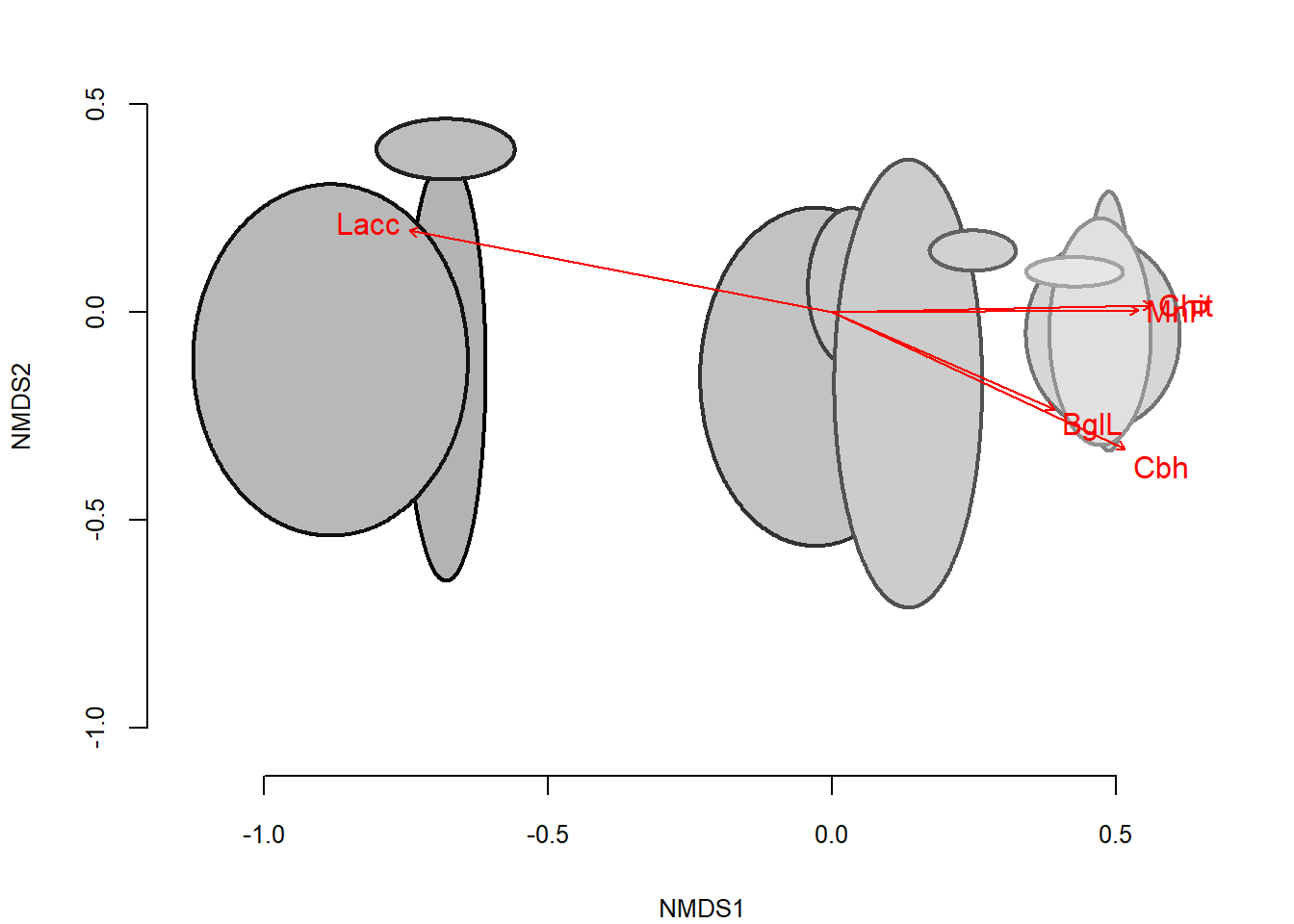# (vii) Finally, for comparison also T-RFLP dataset from 5 oyster mushroom substrate preparation production series (Vajna et al., 2012) was involved, Fig1C # Read final data matrix for relevant samples and selected samples from P. ostreatus substrate preparation production series---- LTS2012_r = read.table(file="L2012_TS_relev Abdo 4_1 merge final.txt",head=T,row.names = 1,sep="\t",dec=".") # Perform NMDS and calculate coordinates for weekly averages---- L_MDS_TS2012<-metaMDS(LTS2012_r[,3:320]) ## Square root transformation ## Wisconsin double standardization ## Run 0 stress 0.1317272 ## Run 1 stress 0.1304945 ## ... New best solution ## ... Procrustes: rmse 0.02068657 max resid 0.09169103 ## Run 2 stress 0.1302853 ## ... New best solution ## ... Procrustes: rmse 0.01467532 max resid 0.0827869 ## Run 3 stress 0.1306495 ## ... Procrustes: rmse 0.01771081 max resid 0.08296646 ## Run 4 stress 0.136818 ## Run 5 stress 0.1350883 ## Run 6 stress 0.130232 ## ... New best solution ## ... Procrustes: rmse 0.01338745 max resid 0.08181182 ## Run 7 stress 0.1304932 ## ... Procrustes: rmse 0.004853079 max resid 0.02266559 ## Run 8 stress 0.1344742 ## Run 9 stress 0.1318968 ## Run 10 stress 0.1368367 ## Run 11 stress 0.1326387 ## Run 12 stress 0.1350567 ## Run 13 stress 0.1301118 ## ... New best solution ## ... Procrustes: rmse 0.01128319 max resid 0.0750981 ## Run 14 stress 0.1373398 ## Run 15 stress 0.1326375 ## Run 16 stress 0.1326101 ## Run 17 stress 0.1302746 ## ... Procrustes: rmse 0.004556023 max resid 0.02782255 ## Run 18 stress 0.1315429 ## Run 19 stress 0.1335164 ## Run 20 stress 0.1375974 ## *** No convergence -- monoMDS stopping criteria: ## 1: no. of iterations >= maxit ## 19: stress ratio > sratmax L_MDS_TS2012_xy <- scores(L_MDS_TS2012, scaling = 2) L_MDS_TS2012_xy[,2]=L_MDS_TS2012_xy[,2]*(-1) L_MDS_TS2012_mean=aggregate(L_MDS_TS2012_xy,list(group=LTS2012_r$Week),mean)
L_MDS_TS2012_mean_TS=L_MDS_TS2012_mean[1:3,]
L_MDS_TS2012_mean_2012=L_MDS_TS2012_mean[4:14,]
L_MDS_TS2012_sd=aggregate(L_MDS_TS2012_xy,list(group=LTS2012_r$Week),sd) L_MDS_TS2012_sd_TS=L_MDS_TS2012_sd[1:3,] L_MDS_TS2012_sd_2012=L_MDS_TS2012_sd[4:14,] # Plotting NMDS for whole dataset---- par(mfrow=c(1,1),mar = c(4,4,4,1),oma=c(0,0,0,0)) plot.new() plot.window(xlim=range(L_MDS_TS2012_xy[,1]), ylim=range(L_MDS_TS2012_xy[,2]), asp = NA) LTS2012_colour=c(gray(5:15/20),rep("white",9),gray(5:15/20)) for (i in 1:length(L_MDS_TS2012_mean_2012[,2])){ plotellipse(rx = L_MDS_TS2012_sd_2012$NMDS1[i], ry = L_MDS_TS2012_sd_2012$NMDS2[i], mid = c(L_MDS_TS2012_mean_2012[i,2],L_MDS_TS2012_mean_2012[i,3]),col=LTS2012_colour[i],lcol=gray(5:15/20)[i],lwd = 0.5)} LTS_colour=c("green2","green3","green4") for (i in 1:length(L_MDS_TS2012_mean_TS[,2])){ plotellipse(rx = L_MDS_TS2012_sd_TS$NMDS1[i], ry = L_MDS_TS2012_sd_TS\$NMDS2[i], mid = c(L_MDS_TS2012_mean_TS[i,2],L_MDS_TS2012_mean_TS[i,3]),lcol=LTS_colour[i],col=NULL)}
# Put data points of selected samples from P. ostreatus substrate preparation production series coded according to stages
points(L_MDS_TS2012_xy[1:15,],cex = 2, pch=16, col=rep(LTS_colour,5)[order(rep(LTS_colour,5),decreasing=F)], bg="black")
# Legend
legend(-3,1.1, c("Stage1","Stage3","Stage7","d1","d71"),pch = 16, col =c(LTS_colour,LTS2012_colour,LTS2012_colour),cex=0.8,bty = "n",horiz = T)
axis(1,cex.axis=0.8)
axis(2,cex.axis=0.8)
title(xlab="NMDS1",ylab="NMDS2",cex.lab=0.8)